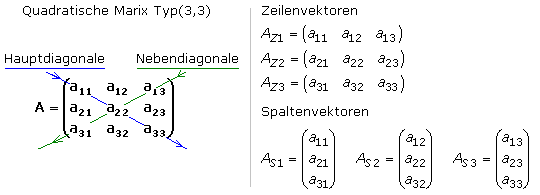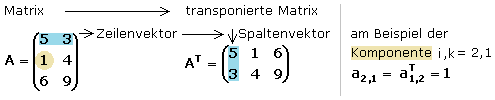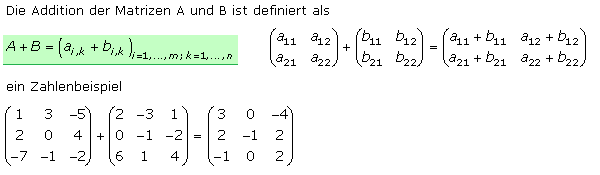# What is row matrix

## Matrices in Mathematics

In the advanced mathematics of linear systems of equations and in electronics, where two-port representations are to be expected in filter circuits, parameters and functions are represented in a special notation, the matrix. The term comes from Latin and stands for a directory as a structured arrangement of data, in biology it refers to a uterus or germinal layer. In the plural it is the matrices.

The matrix is ​​a rectangular system with (m * n) places where the components or elements are arranged in m rows and n columns. Each entry has its defined location index (i, k). The matrix is ​​designated with a capital letter and the component block is placed in round brackets as a whole. In general, the components are given the lower case letter of the matrix identifier with the row and column index. The picture shows the general representation of a matrix.The component ai, k indexed in a matrix A is in the i-th row and k-th column and is thus clearly identified. A matrix with m rows and n columns is of the type (m * n), the rows also being referred to as row vectors and the columns as column vectors of the matrix.

Row matrix
It consists of only one line and is of type (1 * n) and thus a line vector.
Column matrix
It consists of only one column and is of the type (m * 1) and thus a column vector.
Scalar
It is a matrix of the type (1 * 1) with the same row and column vector.

Quadratic matrix

A matrix with the same number of rows and columns is of the type (m * m) and is the only matrix with a main and secondary diagonal. The main diagonal runs from entry a11 at the top left and ends at the bottom right with the entry amm. The secondary diagonal has a comparable course from top right to bottom left. It contains the components a1m and am1. The picture shows the general nomenclature of a square matrix (3,3) and the notation of its row and column vectors.Transposed matrix

If the rows of a matrix A of type (m * n) are written into a new matrix as a column, the transposed matrix A is obtainedT of type (n * m). The components of the matrix and its transposed matrix are related to ai, k = aTk, i. By transposing twice you get the starting matrix again. A square matrix transposed corresponds to the reflection of its components on the main diagonal.Diagonal matrix and identity matrix

It is always a square matrix. With the diagonal matrix, only the main diagonal has values ​​that are not equal to 0. All other components have the value 0. The identity matrix is ​​the special case of a diagonal matrix in which all main diagonal elements have the value 1 and all others have the value 0.

### Arithmetic operations with matrices

There are various arithmetic operations for matrices, only a few of which are briefly presented here. The result is a new matrix.

### Addition of two matrices

The addition is defined for matrices of the same type. When adding, only the components with the same indices are added. The commutative law with A + B = B + A and the associative law with A + (B + C) = (A + B) + C apply. The picture illustrates the addition process.### Subtract two matrices

Only matrices of the same type may be subtracted, whereby the components with the same indices are subtracted from each other. Neither the commutative nor the associative law applies. The following picture shows the general procedure and a numerical example.### Multiplication by a scalar

A matrix can be multiplied by a scalar quantity, a number, with each component being multiplied by the scalar. This also means that if the same scalar value occurs in all components of a matrix, it can be placed in front of the matrix. The associative law and the distributive law apply.### Multiplication of two matrices

The product of matrix A and matrix B only gives result matrix C if the number of columns in matrix A corresponds to the number of rows in matrix B. Matrix A of type (m, n) and matrix B of type (n, p) must therefore be fulfilled, since in matrix multiplication the scalar product is only defined between vectors with the same number of components. The result matrix then has as many rows as matrix A and columns as matrix B. During multiplication, the components are created as scalar products of the i-th row vector of matrix A with the k-th column vector of matrix B. The matrix multiplication allows the associative law and the distributive law, while the commutative law does not apply with a few exceptions.The Falk scheme simplifies the manual multiplication of matrices. The matrices to be multiplied are shifted at right angles to each other so that the rows of matrix A come to lie below and to the left of the columns of matrix B. The crossing points row A * column B can then be easily calculated from the scalar products of the row vector of A with the column vector of B. The video clip shows the Falk scheme.